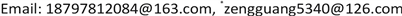1. 引言

f ( P ) = μ ∫ Ω k ( P , Q ) F [ f ( Q ) ] d Q + g ( P ) ,     P , Q ∈ Ω (1)

2. 基于RBF插值改进的配置法算法2.1. RBF插值原理

Φ ( x ) = ∫ ℜ l e i x t Φ ^ ( t ) d t ，当 x j 两两不同且 λ j 不全为零时，有

∑ j , k = 1 n λ j λ k Φ ( ‖ x ‖ ) = ∫ ℜ l | ∑ j n λ j e i x j t | Φ ^ ( t ) d t (2)

(1) ψ ( r ) ∈ C [ 0 , ∞ ] ；

(2) ψ ( r ) ∈ C ∞ [ 0 , ∞ ] ；

(3) ( − 1 ) k ψ k ( r ) > 0 ,   r > 0 ,   k = 0 , 1 , 2 , ⋯ 。

∑ j , k = 1 n λ j λ k Φ ( ‖ x k − x j ‖ ) = ∫ ℜ l | ∑ j = 1 n λ j e i x j t | Φ ^ ( t ) d t > 0 (3)

A = [ Φ ( ‖ x j − x k ‖ ) ( x j β ) ( x j β ) T 0 ]

f * ( x ) = ∑ j = 1 n a j Φ ( ‖ x − x j ‖ ) + ∑ | β | < m b β x β (4)

f ( x ) = f * ( x k ) , k = 1 , 2 , ⋯ , n (5)

2.2. 基于RBF插值的改进配置法算法

RBF是仅依赖于距离 r = ‖ x − x j ‖ 的函数，在多种RBF的定义中，常用的有薄板样条函数 Φ ( r ) = r 2 ln r 高斯函数 Φ ( r ) = e β r 2 、Hardy提岀的多重二次曲面(multi-quadric, MQ)函数及多种紧支撑函数等。Franke在1982年证明了MQ方法是所有插值方法中综合性能最优的，因此本文选用MQ作为插值基函数，其常用表达式为

Φ ( x ) = ‖ x − c ‖ 2 + a 2 (6)

Step 1：设置RBF函数的中心点c，形状参数 α ( α ∈ ( 0 , 1 ] ) ，配置点 x m ,   m = 1 , 2 , ⋯ , N ；

Step 2：对给定的一组数据点 ( x m , f ( x m ) ) ，由RBF构造插值函数

f ( x ) = ∑ i = 1 N λ i Φ i ( x ) = ∑ i = 1 N λ i ( x − c i ) 2 + α 2 (7)

Step 3：将插值点代入(7)式，得

f ( x m ) = ∑ i = 1 N λ i Φ i ( x m ) = ∑ i = 1 N λ i ( x m − c i ) 2 + α 2 (8)

[ Φ d ] [ λ ] = [ f d ] (9)

Step 4: 矩阵元素计算式为 Φ m n = ( x m − c n ) 2 + α 2 ，求解(9)式得

[ λ ] = [ Φ d ] − 1 [ f d ] (10)

Step 5: 得岀函数 f ( x ) 的RBF逼近形式

f ( x ) = [ Φ ( x ) ] [ λ ] = [ Φ ( x ) ] [ Φ d ] − 1 [ f d ] (11)

S ( x ) = ∑ j = 1 n Φ ( ‖ x k − x j ‖ )

S ( x k ) = ∑ j = 1 n λ j Φ ( ‖ x k − x j ‖ ) = f k , k = 1 , 2 , ⋯ , n

∑ ∑ λ j λ k e − ‖ x j − x k ‖ 2 = ( 2 π ) d / 2 ∫ | λ j e i x j θ | 2 e − ‖ θ ‖ 1 / 2 d θ > 0

∑ λ j λ k ∫ 0 ∞ θ − 3 / 2 ( 1 − e − θ ( α 2 + ‖ x ‖ 2 ) ) d θ = ∑ λ j λ k ( α 2 + ‖ x k − x j ‖ 2 ) 1 / 2 ∫ 0 ∞ θ − 3 / 2 ( 1 − e − θ ) d θ = h ∑ λ j λ k ( α 2 + ‖ x k − x j ‖ 2 ) 1 / 2 < 0

∑ λ j λ k ( α 2 + ‖ x k − x j ‖ 2 ) 1 / 2 < 0

∑ λ j λ k ( α 2 + ‖ x k − x j ‖ 2 ) 1 / 2 = ∑ ( α 2 + ‖ x k − x j ‖ 2 ) 1 / 2 > 0

4. 数值实验及分析

L ( x ) = ∑ j = 0 N a j Φ j ( x ) ， (12)

L ( x k ) = ∑ j = 0 N a j Φ j ( x k ) = ∑ j = 0 N a j ( x j − x k ) = f ( x k ) (13)

Φ ( x j − x k ) = α 2 + ( x j − x k ) 2 ( k = 0 , 1 , ⋯ , N ; j = 0 , 1 , ⋯ , N ) 非奇异，故此方程组有唯一解，可求出 a j ，代入(12)式得到 L ( x ) ，进而求出插值函数。下面给出了误差表1及两种插值图1，图2。

The maximum error comparison between the method in this paper and the methods in [<xref ref-type="bibr" rid="hanspub.33077-ref6">6</xref>] [<xref ref-type="bibr" rid="hanspub.33077-ref12">12</xref>] [17
N = 6N = 11

5. 结论